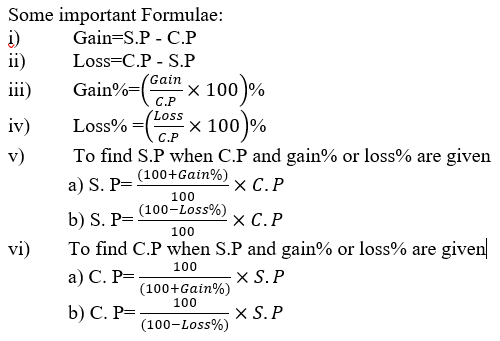# ICSE Worksheet for chapter-8 Profit, Loss and Discount class 9

## Find ICSE Worksheet for chapter-8 Profit, Loss and Discount class 9

CLASS-9

BOARD: ICSE

Mathematic Worksheet - 8

TOPIC: Profit, Loss and Discount

For other ICSE Worksheet for class 9 Mathematic check out main page of Physics Wallah.

#### Definition:

1. Cost price (C.P): The amount for which an article is bought is called its cost price, abbreviated to CP.
2. Selling Price (S.P): The amount for which an article is sold is called its selling price, abbreviated as SP.
3. Gain: When S.P>C.P, then there is a gain. Gain=S.P –C.P
4. Loss: When S.P<C.P, then there is a loss. Loss=C.P-S.P
5.EXERCISE:

1. If the selling price of 20 articles is the same as the cost price of 23 articles, find the profit or loss percent in the transaction.
2. A man buys an article and sells it at a profit of 20%. If he would buy it at 20% less and sell it for Rs.75 less, he would have gained 25%. What is the cost price of the article?
3. The marked price of a woolen coat is Rs.2000. It is sold at a discount of 15%. The shopkeeper has allowed a further discount of 5% due to off season. Find the selling price of the coat.
4. Find a single discount equivalent to the discount series 25%, 20% and 10%.
5. A shopkeeper sells 25 articles at Rs.45 per article after giving 10% discount and earns 50% profit. If the discount is not given, the profit gained is:
1. 60%
2. 60
3. 66%
4. 66
6. The C.P of 21 articles is equal to S.P of 18 articles. Find the gain or loss percent.
1. 16%
2. 8%
3. 10%
4. 12%
7. If the selling price of 50 articles is equal to the cost price of 40 articles, then the loss or gain percent is:
1. 20%gain
2. 20%loss
3. 25%loss
4. 25% gain
8. A trader mixes three varieties of groundnuts costing Rs50, Rs20 and Rs30 per kg in the ratio 2:4:3 in terms of weight, and sells the mixture at Rs33 per kg. What % of profit does he make?
1. 8%
2. 9%
3. 10%
4. None of these
9. Garima purchased a briefcase with an additional 10% discount on the reduced price after deducting 20% on the labeled price. If the labeled price was Rs. 1400, at what price did she purchase the briefcase?
1. Rs. 980
2. Rs. 1008
3. Rs.1056
4. Rs. 1120
10. A shopkeeper sells a badminton racket, whose marked price is Rs. 30, at a discount of 15% and gives a shuttle cock costing Rs. 1.50 free with each racket. Even then he makes a profit of 20%. His C.P per racket is:
1. Rs. 19.75
2. Rs. 20
3. Rs. 21
4. Rs. 21.25

1. Profit 15%
2. Rs.375
3. Rs.1615
4. 46%
5. (d)
6. (a)
7. (b)
8. (c)
9. (b)
10. (b)